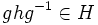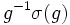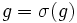# Normal and centralizer-free implies automorphism-faithful

This article gives the statement and possibly, proof, of an implication relation between two subgroup properties. That is, it states that every subgroup satisfying the first subgroup property (i.e., centralizer-free normal subgroup) must also satisfy the second subgroup property (i.e., automorphism-faithful normal subgroup)
View all subgroup property implications | View all subgroup property non-implications
Get more facts about centralizer-free normal subgroup|Get more facts about automorphism-faithful normal subgroup

## Statement

Suppose$H$ is a subgroup of a group$G$ such that:

•$H$ is centralizer-free in$G$: The centralizer$C_G(H)$ is trivial.
•$H$ is normal in$G$.

Then,$H$ is an Automorphism-faithful subgroup (?) of$G$: every nontrivial automorphism of$G$ that restricts to$H$ must restrict to a nontrivial automorphism of$H$.

## Facts used

1. Three subgroup lemma

## Proof

### Hands-on proof

Given: A group$G$, a normal subgroup$H$ such that$C_G(H)$ is trivial. An automorphism$\sigma$ of$G$ that acts as the identity on$H$.

To prove:$\sigma$ is the identity automorphism.

Proof: Note that for$g \in G, h \in H$, we have, by normality, that$ghg^{-1} \in H$. Thus, since$\sigma$ acts as the identity on$H$, we get:$\sigma(ghg^{-1}) = ghg^{-1}$.

Expand the left side and use that$\sigma(h) = h$ to obtain:$\sigma(g)h\sigma(g)^{-1} = ghg^{-1}$.

Rearranging this yields that$g^{-1}\sigma(g)$ commutes with$h$. Since this holds true for every$h \in H$, we obtain that$g^{-1}\sigma(g) \in C_G(H)$. Since$C_G(H)$ is trivial, we obtain that for every$g \in G$,$g^{-1}\sigma(g)$ is the identity element, yielding$g = \sigma(g)$ for all$g \in G$. This yields that$\sigma$ is the identity automorphism.

### Proof using three subgroup lemma

Note that although this proof may superficially appear different from the preceding one, it is a less hands-on formulation of the same proof. This style of proof may be more useful when proving similar statements that are more complicated; for instance: normal and self-centralizing implies coprime automorphism-faithful.

Given: A group$G$, a normal subgroup$H$ such that$C_G(H)$ is trivial. An automorphism$\sigma$ of$G$ that acts as identity on$H$.

To prove:$\sigma$ is the identity automorphism.

Proof: Suppose$A$ is the subgroup generated by$\sigma$ in$\operatorname{Aut}(G)$, and let's assume we're working in$G \rtimes A$. By assumption,$[H,A]$ is trivial. Since$H$ is normal in$G$, we have$[G,H] \le H$. Thus, we have:

•$[[G,H],A] \le [H.A]$, which is trivial, so$[[G,H],A]$ is trivial
•$[[H,A],G]$ is trivial, because$[H,A]$ is trivial

So, by fact (1) (the three subgroup lemma),$[[G,A],H]$ is trivial. Since$[G,A] \le G$, we see that$[G,A] \le C_G(H)$, so by the assumption of$C_G(H)$ being trivial, we obtain that$[G,A]$ is trivial. This translates to saying that every element of$A$ fixes every element of$G$, so$\sigma$ must be the identity automorphism.